Next: 5. Kernel PCA for Up: 4. Abstract recurrent neural Previous: 4.6 Dependence on the

# 4.7 Discussion

A pattern association model, called abstract recurrent neural network, was introduced that works analogue to an RNN. Like feed-forward networks, the model can be trained on any data distribution. It has, however, two advantages over feed-forward networks. First, a trained abstract RNN can associate patterns in any mapping direction. Second, the model can cope with one-to-many mappings.

The recall algorithm works on top of a mixture of local PCA (geometrically a mixture of ellipsoids) that approximates the data distribution. Different from a gradient descent relaxation, the algorithm avoids local minimum since an analytical solution exists that maps an input pattern directly onto its completion. The algorithm is further independent of the method that produced the mixture model, and in all examples from this chapter, also the recall results were similar for different local PCA methods.

The mapping obtained by the abstract RNN is locally linear. At the transition between two ellipsoids the mapping is discontinuous (figure 4.3 and 4.11). Avoiding these discontinuities might improve the algorithm. One possibility may be to interpolate between neighboring ellipsoids. Here, however, the difficulty is to find a neighbor that can continue the solution instead of providing an alternative solution (interpolating between alternative solutions leads to errors). Figure 4.20 shows a possible problem. The neighbor ellipse with the alternative solution can have the smallest Euclidean and Mahalanobis distance to the current ellipse.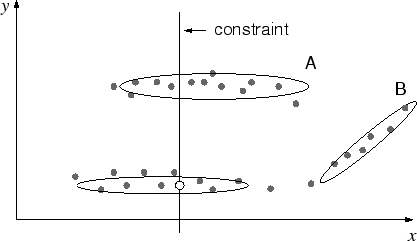Tavan et al. (1990) suggested another recall algorithm based on a density model of the training patterns. Here, the density is a mixture of uniform Gaussian functions. Recall happens in a recurrent radial basis function network. Its activation functions are the Gaussians from the local densities. Thus, the current state is a weighted sum of the Gaussian centers. To avoid local minima, the algorithm anneals (shrinks) the width of the Gaussians parallel to the recurrent state update. For the constrained recall, however, a straight-forward extension of this algorithm to ellipsoids did not have a comparable performance as the abstract RNN (figure 4.21). The extension consists of three parts: the uniform Gaussians are replaced by multi-variate ones (2.16); the updated state is projected onto the constrained space, and the annealing is realized by coupling all eigenvalues to a global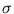value (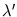=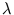+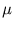(-) with the coupling;is kept constant, whileslowly decreases during the annealing). The completion did not follow the shape of the tilted ellipses because the weighted sum of centers came out to be the center of only one ellipse; if a state is close enough to one ellipse, the activation of the other ellipses can be neglected.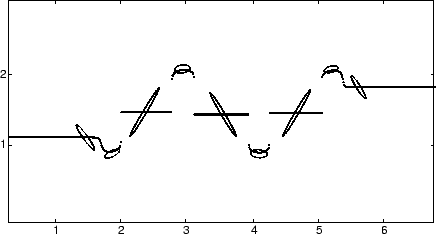The abstract RNN could be successfully applied to the completion of images (section 4.4). In comparison, a discrete or continuous Hopfield network (Hopfield, 1982,1984) cannot be used to recall gray-scaled images. On the face completion task, the abstract RNN was even better then a table look-up on the whole training set.

On the completion of small windows, the mixture of local PCA resulted in about the same recall error as a single unit. With both single and mixture model, the recalled images seem to capture only the low-pass filtered image part. They are averages over all windows that match the input pixels. Moreover, the midpoints of the mixture model came out to be just monotone gray tones. Apparently, the distribution of images just extends from the origin into different subspaces. Thus, the advantage of having different unit centers is lost.

On the faces, the mixture model was better than a single unit if large connected areas needed to be filled. Here, multiple solutions can exists for a given input, and the mixture model could cover the different solutions. However, if only thin stripes needed to be filled, the solution is not ambiguous, and here, the single unit was better.

The abstract RNN could learn the kinematics of a robot arm (section 4.5). The inverse direction had redundant degrees of freedom. Nevertheless, the model could recall a posture that brought the end-effector to a given position; a multi-layer perceptron could not. All mixture of local PCA variants did almost equally well. In this experiment, NGPCA did not produce dead units (units with no patterns assigned to); the smallest number of patterns for one unit was around 50 (figure 4.10). This possibly explains why NGPCA-constV did not bring an improvement (see section 3.2.2). The slight decrease in performance for NGPCA-constV might result from ellipsoids that erroneously connect distant parts of the distribution, as observed in figure 3.8.C. These ellipsoids have only a few assigned patterns, and this might explain, why NGPCA-constV results in a lower minimal number of assigned patterns per unit (figure 4.10).

The following rules can be given for the number of units m and principal components q. Increasing m increased the performance because the training patterns lie on a manifold that is non-linear. A stable training, however, limits the maximum number of units (this depends on the number of training patterns). The optimal number of principal components was equal to the local dimensionality of the distribution. For arbitrary distributions, the local dimensionality can be obtained by computing a PCA on a local neighborhood within the distribution (section 4.5.1 and figure 4.12, left).

For an optimal performance, it was expected that the local dimensionality sets the minimum value for q because fewer principal components cannot describe the local extend of the distribution. However, it was not expected that further increasing q decreased the performance. An explanation might be that the eigenvalues in the direction orthogonal to the kinematic manifold were unequal (figure 4.12, left). Thus, for q > 6 the error measure (4.1) is not isotropic for directions orthogonal to the manifold (as it should be ideally), and this may disturb the competition between the units in the recall algorithm.

The abstract RNN could cope with additional noise dimensions. If they are added, the pattern distribution also extends to these dimensions. Thus, the local dimensionality increases by the number of added noise dimensions, and q needs to be adjusted accordingly to get the same performance.

The mean error of the recall increased with the number r of input dimensions given a constant m. This increase was observed in the experiment with the kinematic arm model and in a simplified stochastic version of the abstract RNN. Both tests could produce an exponential increase for intermediate values of r (figure 4.13 and 4.19). Moreover, the exponent was of the same order (0.59 in experiment compared to 0.69 in theory), despite the rough simplifications made in the theory. Thus, the increase in error with increasing r is possibly a characteristic of the recall algorithm. Therefore, in applications, mappings from many dimensions to only a few (for example, ten to two) should be avoided.

In chapter 6 the abstract RNN is applied to a real robot arm. There, the robot's task is to grasp an object, and the RNN associates an arm posture with an image of the object.Next: 5. Kernel PCA for Up: 4. Abstract recurrent neural Previous: 4.6 Dependence on the
Heiko Hoffmann
2005-03-22